# Branching process with a random medium

(diff) ← Older revision | Latest revision (diff) | Newer revision → (diff)

branching process in a random medium

A time-inhomogeneous branching process in which the inhomogeneity is random. Let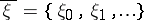be a stationary sequence of random variables (the value ofis interpreted as the state of the "medium" at the moment of time), and let to each possible state of the medium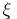correspond a probability distribution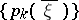of the number of descendants of a single particle: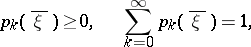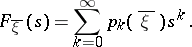In order to construct a trajectoryof a branching process in a random medium the value of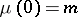and the trajectoryof the states of the medium are fixed, and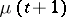is determined for each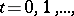as a sum of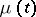independent random variables with distribution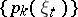. Such a complication of the branching Galton–Watson process is natural if, for example, the branching process in a random medium is regarded as a model of a biological population.

The properties of branching processes in a random medium are analogous to those of ordinary branching processes. For instance, the generating distribution function of, under the condition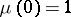, has the form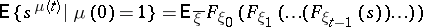(*)

(for a branching Galton–Watson process, i.e. for, the right-hand side of (*) equals the-fold iteration of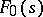). Branching processes in a random medium may be subcritical, critical and supercritical: the "criticality parameter" here (see ) is the variable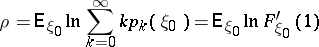(for ordinary branching processes, the "criticality parameter" is the mathematical expectation of the number of "descendants" of a single particle). If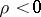, the branching process in a random medium is said to be subcritical and, for the random variable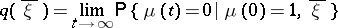which is the probability of extinction of the branching process in a random medium for a given trajectory, the relation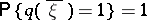is valid. There is also the analogue of the limit theorem of the subcritical Galton–Watson branching process: For almost all realizations of the sequencethe limits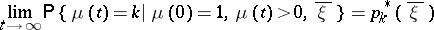exist and satisfy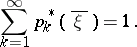If, the branching process in a random medium is said to be critical, andand, for almost all realizations of,If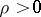, the branching process in a random medium is said to be supercritical; in such a case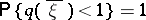and, if certain additional conditions are met, there exists for almost alla non-negative random variable,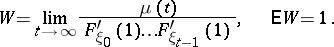How to Cite This Entry:
Branching process with a random medium. Encyclopedia of Mathematics. URL: http://encyclopediaofmath.org/index.php?title=Branching_process_with_a_random_medium&oldid=13341
This article was adapted from an original article by A.M. Zubkov (originator), which appeared in Encyclopedia of Mathematics - ISBN 1402006098. See original article